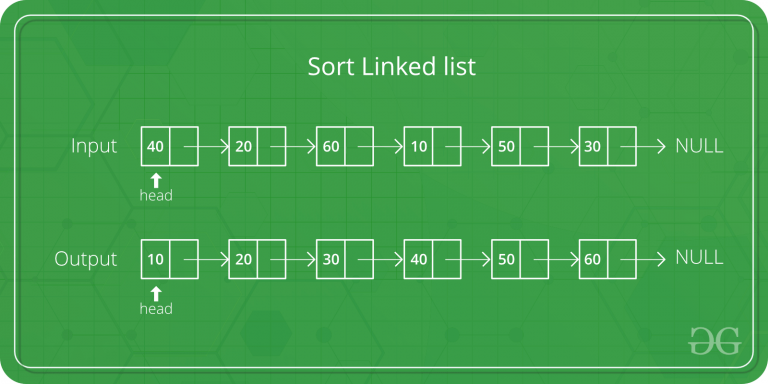# Java Program for Merge Sort for Linked Lists

Merge sort is often preferred for sorting a linked list. The slow random-access performance of a linked list makes some other algorithms (such as quicksort) perform poorly, and others (such as heapsort) completely impossible.Let head be the first node of the linked list to be sorted and headRef be the pointer to head. Note that we need a reference to head in MergeSort() as the below implementation changes next links to sort the linked lists (not data at the nodes), so head node has to be changed if the data at original head is not the smallest value in linked list.

```MergeSort(headRef)
1) If head is NULL or there is only one element in the Linked List
then return.
2) Else divide the linked list into two halves.
FrontBackSplit(head, &a, &b); /* a and b are two halves */
3) Sort the two halves a and b.
MergeSort(a);
MergeSort(b);
4) Merge the sorted a and b (using SortedMerge() discussed here)
```

## Java

 `// Java program to illustrate merge sorted ` `// of linkedList ` ` `  `public` `class` `linkedList { ` `    ``node head = ``null``; ` `    ``// node a, b; ` `    ``static` `class` `node { ` `        ``int` `val; ` `        ``node next; ` ` `  `        ``public` `node(``int` `val) ` `        ``{ ` `            ``this``.val = val; ` `        ``} ` `    ``} ` ` `  `    ``node sortedMerge(node a, node b) ` `    ``{ ` `        ``node result = ``null``; ` `        ``/* Base cases */` `        ``if` `(a == ``null``) ` `            ``return` `b; ` `        ``if` `(b == ``null``) ` `            ``return` `a; ` ` `  `        ``/* Pick either a or b, and recur */` `        ``if` `(a.val <= b.val) { ` `            ``result = a; ` `            ``result.next = sortedMerge(a.next, b); ` `        ``} ` `        ``else` `{ ` `            ``result = b; ` `            ``result.next = sortedMerge(a, b.next); ` `        ``} ` `        ``return` `result; ` `    ``} ` ` `  `    ``node mergeSort(node h) ` `    ``{ ` `        ``// Base case : if head is null ` `        ``if` `(h == ``null` `|| h.next == ``null``) { ` `            ``return` `h; ` `        ``} ` ` `  `        ``// get the middle of the list ` `        ``node middle = getMiddle(h); ` `        ``node nextofmiddle = middle.next; ` ` `  `        ``// set the next of middle node to null ` `        ``middle.next = ``null``; ` ` `  `        ``// Apply mergeSort on left list ` `        ``node left = mergeSort(h); ` ` `  `        ``// Apply mergeSort on right list ` `        ``node right = mergeSort(nextofmiddle); ` ` `  `        ``// Merge the left and right lists ` `        ``node sortedlist = sortedMerge(left, right); ` `        ``return` `sortedlist; ` `    ``} ` ` `  `    ``// Utility function to get the middle of the linked list ` `    ``node getMiddle(node h) ` `    ``{ ` `        ``// Base case ` `        ``if` `(h == ``null``) ` `            ``return` `h; ` `        ``node fastptr = h.next; ` `        ``node slowptr = h; ` ` `  `        ``// Move fastptr by two and slow ptr by one ` `        ``// Finally slowptr will point to middle node ` `        ``while` `(fastptr != ``null``) { ` `            ``fastptr = fastptr.next; ` `            ``if` `(fastptr != ``null``) { ` `                ``slowptr = slowptr.next; ` `                ``fastptr = fastptr.next; ` `            ``} ` `        ``} ` `        ``return` `slowptr; ` `    ``} ` ` `  `    ``void` `push(``int` `new_data) ` `    ``{ ` `        ``/* allocate node */` `        ``node new_node = ``new` `node(new_data); ` ` `  `        ``/* link the old list off the new node */` `        ``new_node.next = head; ` ` `  `        ``/* move the head to point to the new node */` `        ``head = new_node; ` `    ``} ` ` `  `    ``// Utility function to print the linked list ` `    ``void` `printList(node headref) ` `    ``{ ` `        ``while` `(headref != ``null``) { ` `            ``System.out.print(headref.val + ``" "``); ` `            ``headref = headref.next; ` `        ``} ` `    ``} ` ` `  `    ``public` `static` `void` `main(String[] args) ` `    ``{ ` ` `  `        ``linkedList li = ``new` `linkedList(); ` `        ``/* ` `         ``* Let us create a unsorted linked lists to test the functions Created ` `         ``* lists shall be a: 2->3->20->5->10->15 ` `         ``*/` `        ``li.push(``15``); ` `        ``li.push(``10``); ` `        ``li.push(``5``); ` `        ``li.push(``20``); ` `        ``li.push(``3``); ` `        ``li.push(``2``); ` `        ``System.out.println(``"Linked List without sorting is :"``); ` `        ``li.printList(li.head); ` ` `  `        ``// Apply merge Sort ` `        ``li.head = li.mergeSort(li.head); ` `        ``System.out.print(``"\n Sorted Linked List is: \n"``); ` `        ``li.printList(li.head); ` `    ``} ` `} ` ` `  `// This code is contributed by Rishabh Mahrsee `

Output:

```Linked List without sorting is :
2 3 20 5 10 15
2 3 5 10 15 20
```

Please refer complete article on Merge Sort for Linked Lists for more details!

My Personal Notes arrow_drop_up
Article Tags :

1

Please write to us at contribute@geeksforgeeks.org to report any issue with the above content.